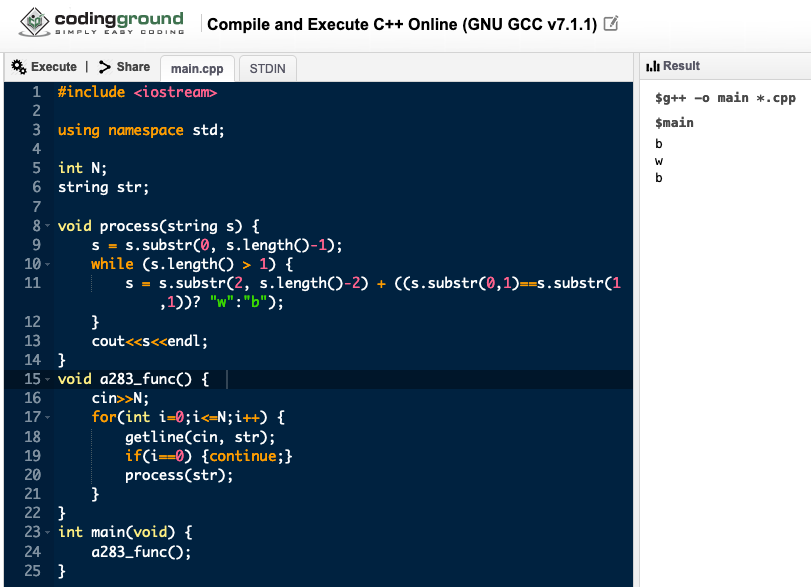#2

## 拜託求救

3
wbw
bwwb
wwbwwbb

b
w
b

### 4 個回答

1``````#include <iostream>

using namespace std;

int N;
string str;

void process(string s) {
s = s.substr(0, s.length()-1);
while (s.length() > 1) {
s = s.substr(2, s.length()-2) + ((s.substr(0,1)==s.substr(1,1))? "w":"b");
}
cout<<s<<endl;
}
void a283_func() {
cin>>N;
for(int i=0;i<=N;i++) {
getline(cin, str);
if(i==0) {continue;}
process(str);
}
}
int main(void) {
a283_func();
}
``````
4

``````numCase = int(input(''))

for i in range(numCase):
series = input('')

while len(series) > 1:
if series == series:
series += 'w'
else:
series += 'b'

series = series[2:]

print(series)
``````